Question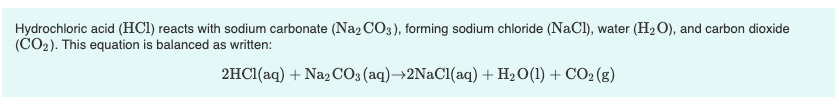1. What volume of 1.00 M HCl in liters is needed to react completely (with nothing left over) with 0.750 L of 0.200 M Na2CO3?

Express your answer to three significant figures and include the appropriate units.

2. A 639-mL sample of unknown HCl solution reacts completely with Na2CO3 to form 15.1 g CO2. What was the concentration of the HCl solution?

Express your answer to three significant figures and include the appropriate units.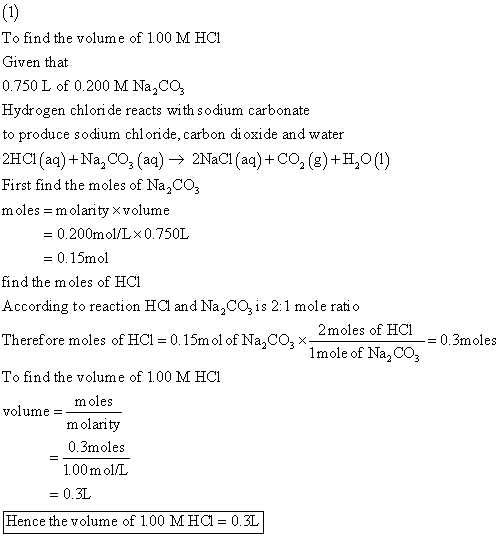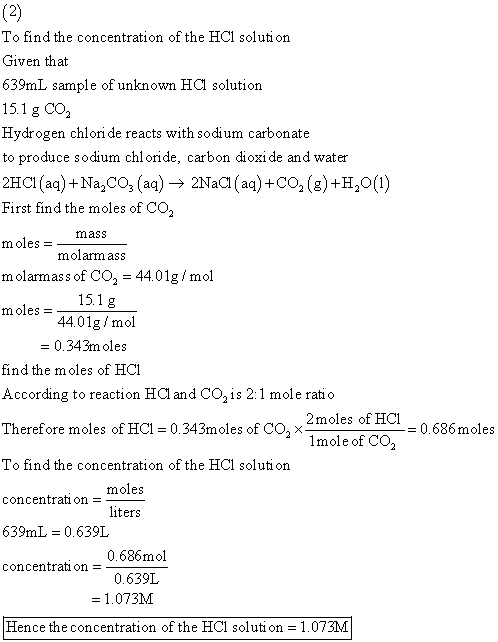#### Earn Coins

Coins can be redeemed for fabulous gifts.

Similar Homework Help Questions
• ### Part A What volume of 1.00 M HCl in liters is needed to react completely (with...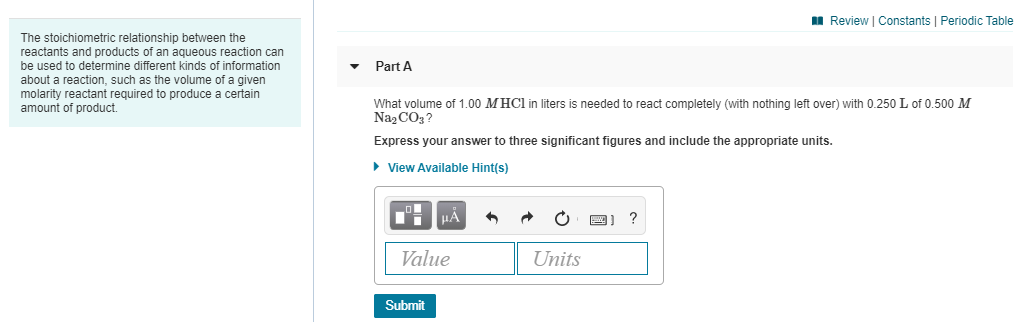Part A What volume of 1.00 M HCl in liters is needed to react completely (with nothing left over) with 0.250 L of 0.500 M Na2CO3? Express your answer to three significant figures and include the appropriate units. Part B A 419-mL sample of unknown HCl solution reacts completely with Na2CO3 to form 12.1 g CO2. What was the concentration of the HCl solution? Express your answer to three significant figures and include the appropriate units. A Review Constants Periodic...

• ### 1. What is the final volume in milliliters when 0.653 L of a 45.4 % (m/v)...

1. What is the final volume in milliliters when 0.653 L of a 45.4 % (m/v) solution is diluted to 24.0 % (m/v)? Express your answer with the appropriate units. 2. A 751 mL NaCl solution is diluted to a volume of 1.06 L and a concentration of 8.00 M . What was the initial concentration? Express your answer with the appropriate units. 3. What volume of 1.00 M HCl in liters is needed to react completely (with nothing left...

• ### 4. The stoichiometric relationship between the reactants and products of an aqueous reaction can be used...

4. The stoichiometric relationship between the reactants and products of an aqueous reaction can be used to determine different kinds of information about a reaction, such as the volume of a given molarity reactant required to produce a certain amount of product. Hydrochloric acid (HCl) reacts with sodium carbonate (Na2CO3), forming sodium chloride (NaCl), water (H2O), and carbon dioxide (CO2). This equation is balanced as written: 2HCl(aq)+Na2CO3(aq)→2NaCl(aq)+H2O(l)+CO2(g) A.) What volume of 3.00 M HCl in liters is needed to react...

• ### The stoichiometric relationship between the reactants and products of an aqueous reaction can be used to...

The stoichiometric relationship between the reactants and products of an aqueous reaction can be used to determine different kinds of information about a reaction, such as the volume of a given molarity reactant required to produce a certain amount of product. Hydrochloric acid (HCl) reacts with sodium carbonate (Na2CO3), forming sodium chloride (NaCl), water (H2O), and carbon dioxide (CO2). This equation is balanced as written: 2HCl(aq)+Na2CO3(aq)→2NaCl(aq)+H2O(l)+CO2(g) a. What volume of 2.50 M HCl in liters is needed to react completely...

• ### Solution Stoichiometry Hydrochloric acid (HCl) reacts with sodium carbonate (Na2CO3), forming sodium chloride (NaCl), water (H2O),...

Solution Stoichiometry Hydrochloric acid (HCl) reacts with sodium carbonate (Na2CO3), forming sodium chloride (NaCl), water (H2O), and carbon dioxide (CO2). This equation is balanced as written: 2HCl(aq)+Na2CO3(aq)→2NaCl(aq)+H2O(l)+CO2(g) a) What volume of 2.75 M HCl in liters is needed to react completely (with nothing left over) with 0.750 L of 0.300 M Na2CO3? b) A 565-mL sample of unknown HCl solution reacts completely with Na2CO3 to form 10.1 g CO2. What was the concentration of the HCl solution? How do I...

• ### Part A What volume of 1.75 M HCl in liters is needed to react completely (with...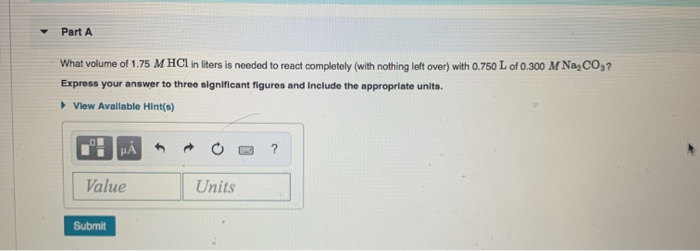Part A What volume of 1.75 M HCl in liters is needed to react completely (with nothing left over) with 0.750 L of 0.300 M NaCO3? Express your answer to three significant figures and include the appropriate units. View Available Hint(s) I HẢ * * 0 -3 ? Value Units Submit

• ### The stoichiometric relationship between the reactants and products of an aqueous reaction can be used to...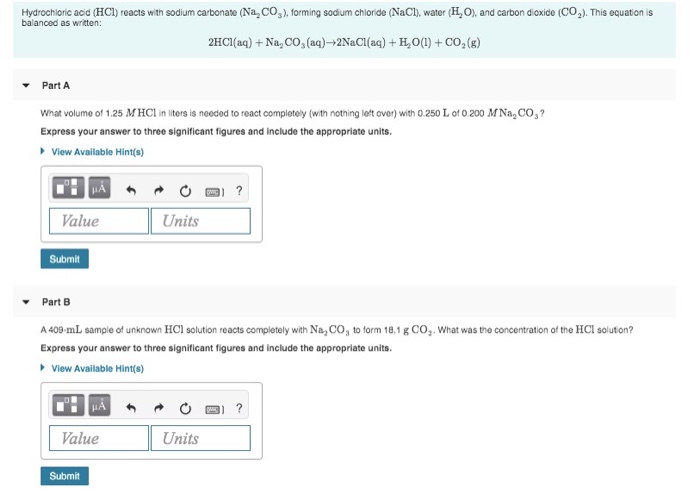The stoichiometric relationship between the reactants and products of an aqueous reaction can be used to determine different kinds of information about a reaction, such as the volume of a given molarity reactant required to produce a certain amount of product. Hydrochloric acid (HCl) reacts with sodium carbonate (Na CO2). forming sodium chloride (NaCl), water (HO), and carbon dioxide (C0.). This equation is balanced as written: 2HCl(aq) + Na, CO, (aq)-2NaCl(aq) + H,0(1) + CO2(g) Part A What volume of...

• ### WOL 2. What volume of 0.250 M HCl is required to completely react with 0.350 g of Na2CO3? Na2CO3(s) + 2HCl(aq) + 2N...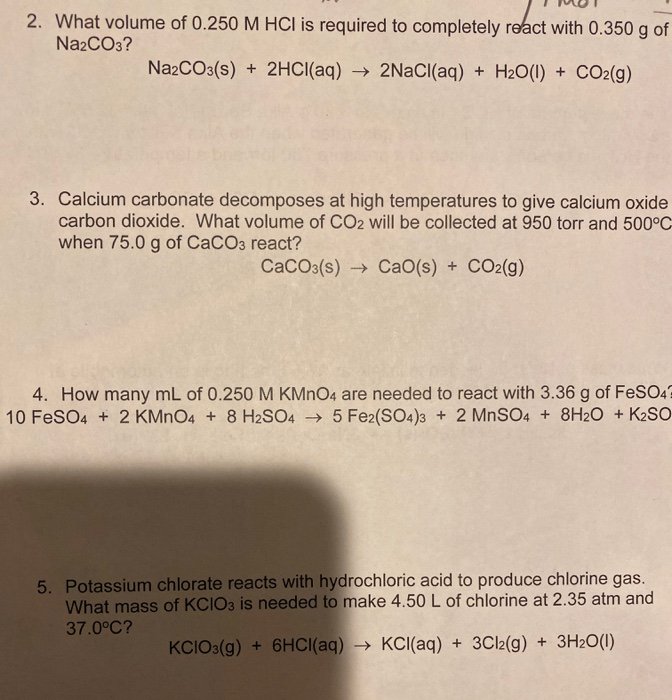WOL 2. What volume of 0.250 M HCl is required to completely react with 0.350 g of Na2CO3? Na2CO3(s) + 2HCl(aq) + 2NaCl(aq) + H2O(l) + CO2(g) 3. Calcium carbonate decomposes at high temperatures to give calcium oxide carbon dioxide. What volume of CO2 will be collected at 950 torr and 500°C when 75.0 g of CaCO3 react? CaCO3(s) → Cao(s) + CO2(g) 4. How many mL of 0.250 M KMnO4 are needed to react with 3.36 g of FeSO4...

• ### Calcium carbonate (CaCO3) reacts with stomach acid (HCl, hydrochloric acid) according to the following equation: CaCO3(s)...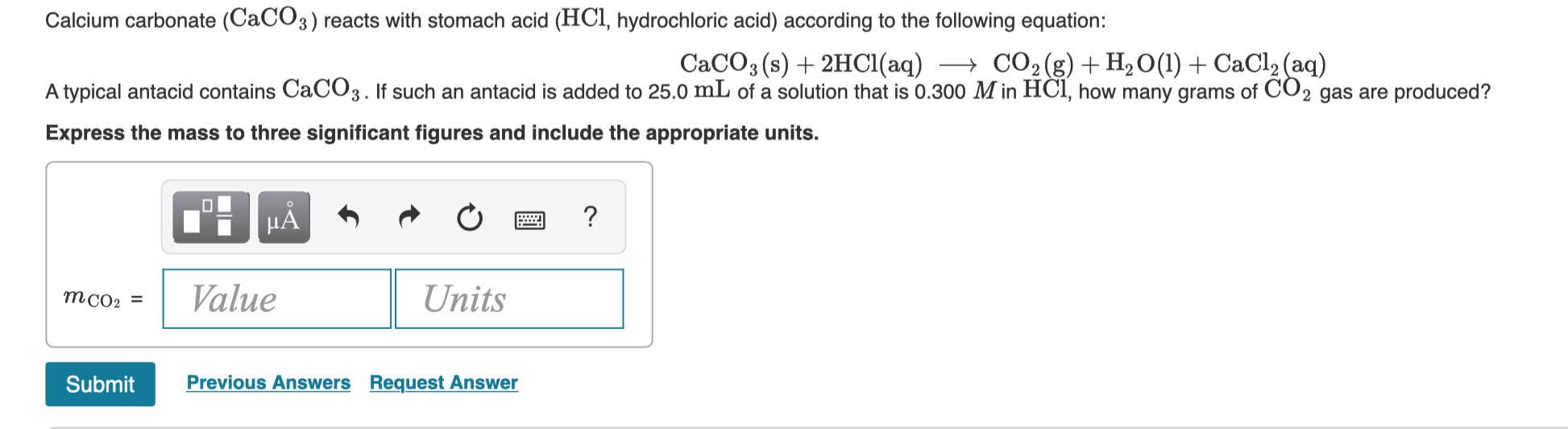Calcium carbonate (CaCO3) reacts with stomach acid (HCl, hydrochloric acid) according to the following equation: CaCO3(s) + 2HCl(aq) + CO2(g) + H2O(l) + CaCl2(aq) A typical antacid contains CaCO3. If such an antacid is added to 25.0 mL of a solution that is 0.300 M in HCl, how many grams of CO2 gas are produced? Express the mass to three significant figures and include the appropriate units. TI MÃ + + + a ? Value Units MCO: = Submit Previous...

• ### Determine the volume of 0.170 M NaOH solution required to neutralize each sample of hydrochloric acid....

Determine the volume of 0.170 M NaOH solution required to neutralize each sample of hydrochloric acid. The neutralization reaction is: NaOH(aq)+HCl(aq)→H2O(l)+NaCl(aq) 45 mL of a 0.050 M HCl solution Express your answer to two significant figures and include the appropriate units. 165 mL of a 0.885 M HCl solution Express your answer to three significant figures and include the appropriate units.